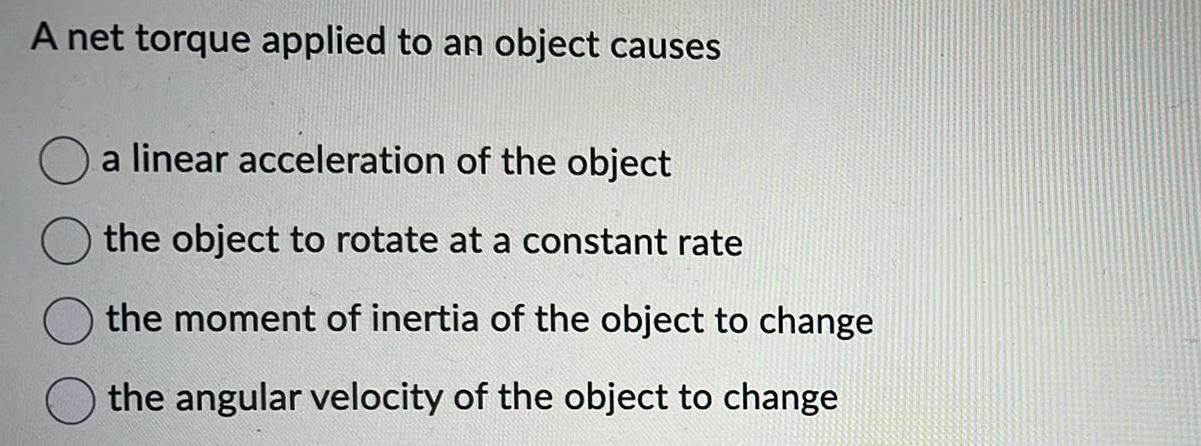Physics
Rotation
A net torque applied to an object causes a linear acceleration of the object the object to rotate at a constant rate the moment of inertia of the object to change the angular velocity of the object to change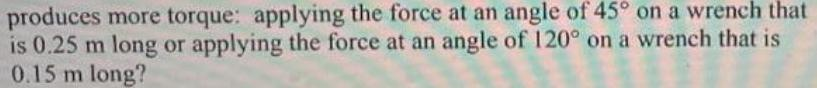Physics
Rotation
produces more torque: applying the force at an angle of 45° on a wrench that is 0.25 m long or applying the force at an angle of 120° on a wrench that is 0.15 m long?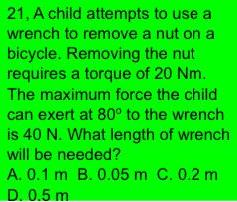Physics
Rotation
A child attempts to use a wrench to remove a nut on a bicycle. Removing the nut requires a torque of 20 Nm. The maximum force the child can exert at 80° to the wrench is 40 N. What length of wrench will be needed? A. 0.1 m B. 0.05 m C. 0.2 m D. 0.5 m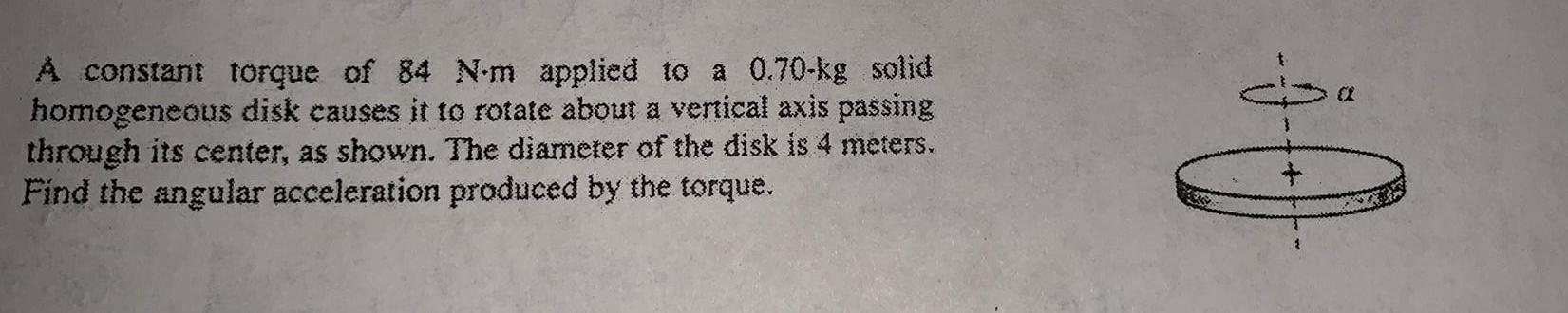Physics
Rotation
A constant torque of 84 N-m applied to a 0.70-kg solid homogeneous disk causes it to rotate about a vertical axis passing through its center, as shown. The diameter of the disk is 4 meters. Find the angular acceleration produced by the torque.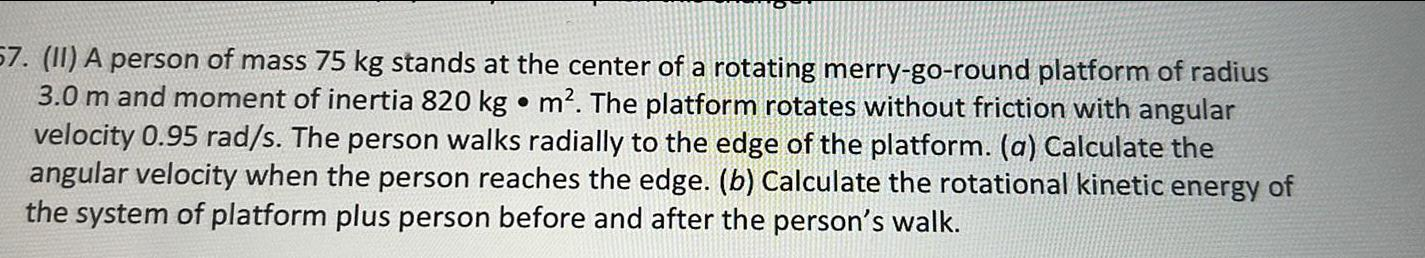Physics
Rotation
57. (II) A person of mass 75 kg stands at the center of a rotating merry-go-round platform of radius 3.0 m and moment of inertia 820 kg m². The platform rotates without friction with angular velocity 0.95 rad/s. The person walks radially to the edge of the platform. (a) Calculate the angular velocity when the person reaches the edge. (b) Calculate the rotational kinetic energy of the system of platform plus person before and after the person's walk.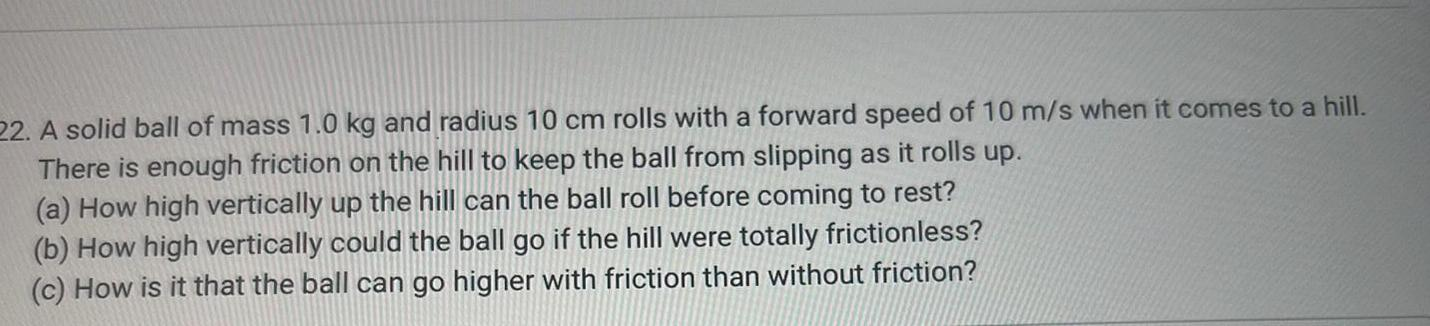Physics
Rotation
22. A solid ball of mass 1.0 kg and radius 10 cm rolls with a forward speed of 10 m/s when it comes to a hill. There is enough friction on the hill to keep the ball from slipping as it rolls up. (a) How high vertically up the hill can the ball roll before coming to rest? (b) How high vertically could the ball go if the hill were totally frictionless? (c) How is it that the ball can go higher with friction than without friction?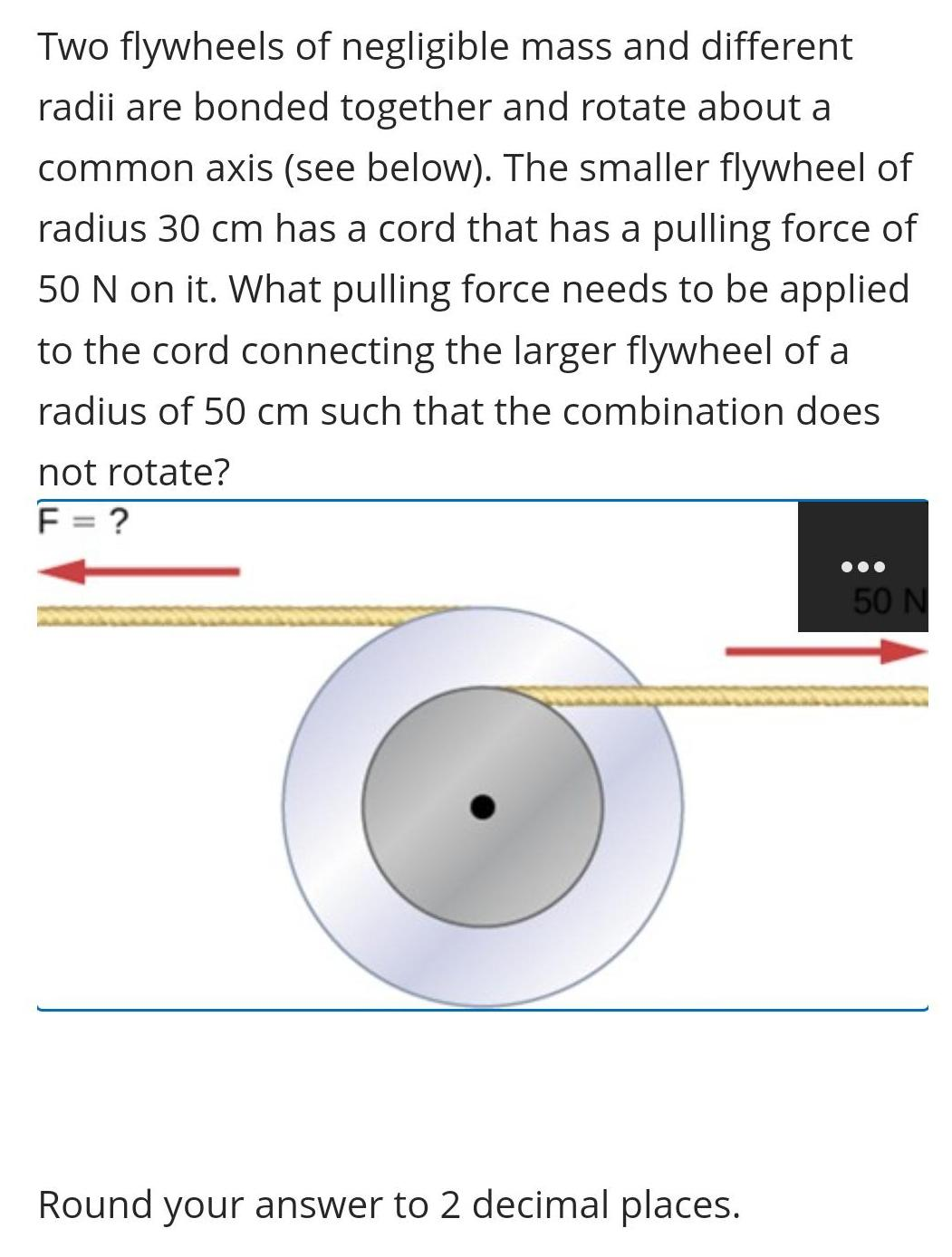Physics
Rotation
Two flywheels of negligible mass and different radii are bonded together and rotate about a common axis (see below). The smaller flywheel of radius 30 cm has a cord that has a pulling force of 50 N on it. What pulling force needs to be applied to the cord connecting the larger flywheel of a radius of 50 cm such that the combination does not rotate? F = ? Round your answer to 2 decimal places.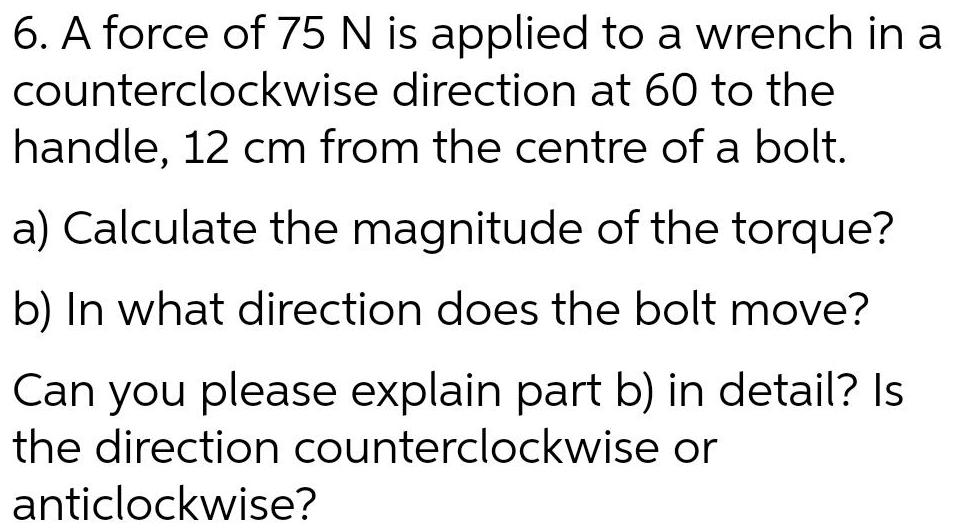Physics
Rotation
6. A force of 75 N is applied to a wrench in a counterclockwise direction at 60 to the handle, 12 cm from the centre of a bolt. a) Calculate the magnitude of the torque? b) In what direction does the bolt move? Can you please explain part b) in detail? Is the direction counterclockwise or anticlockwise?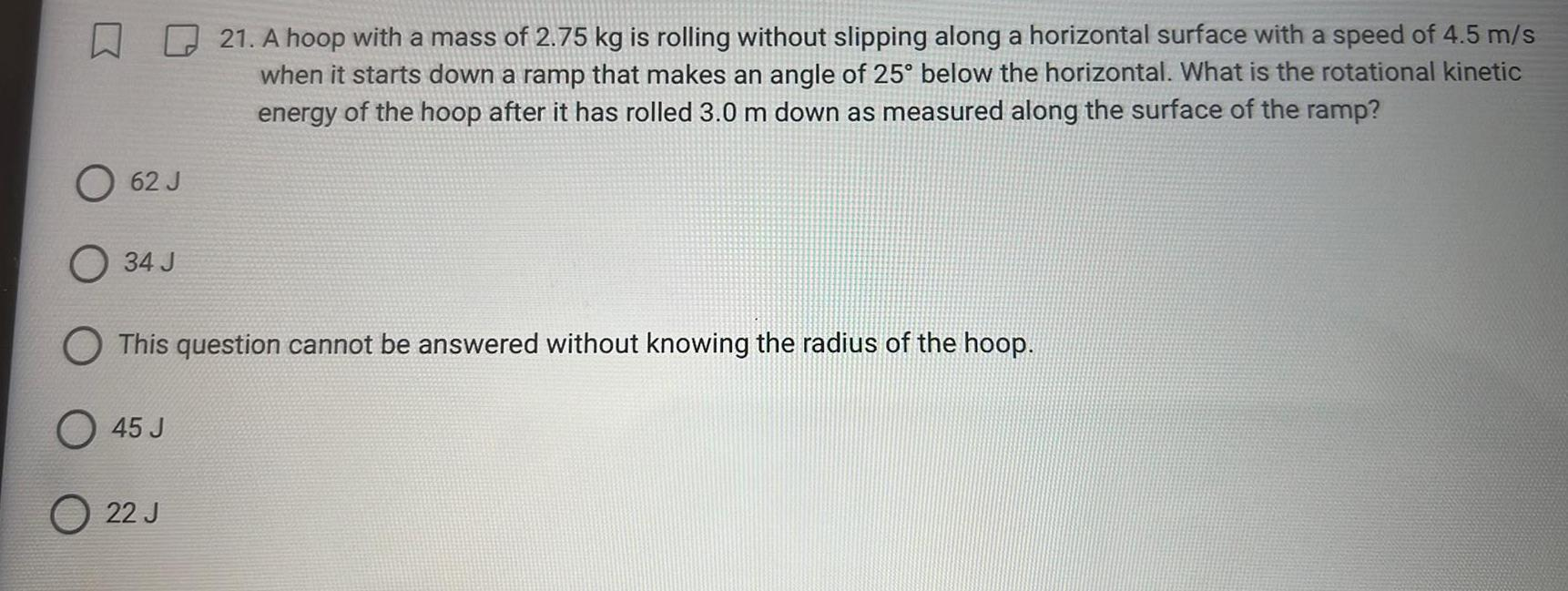Physics
Rotation
21. A hoop with a mass of 2.75 kg is rolling without slipping along a horizontal surface with a speed of 4.5 m/s when it starts down a ramp that makes an angle of 25° below the horizontal. What is the rotational kinetic energy of the hoop after it has rolled 3.0 m down as measured along the surface of the ramp? O 62 J O 34 J O This question cannot be answered without knowing the radius of the hoop. O 45 J O 22 J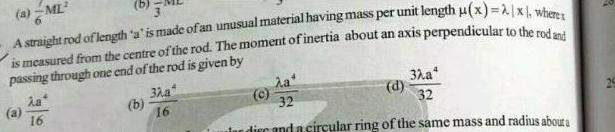Physics
Rotation
A straight rod of length 'a' is made of an unusual material having mass per unit length u(x)=2x, where is measured from the centre of the rod. The moment of inertia about an axis perpendicular to the rod and passing through one end of the rod is given by (a)λa4/16 (b)3λa4/16 (c)λa4/32 (d)3λa4/32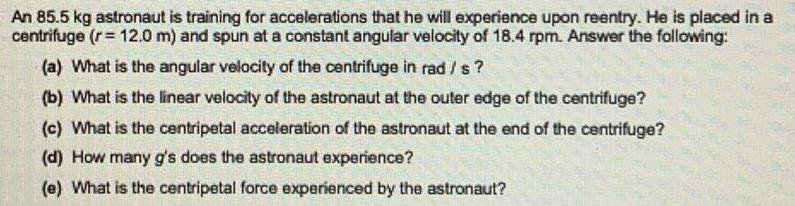Physics
Rotation
An 85.5 kg astronaut is training for accelerations that he will experience upon reentry. He is placed in a centrifuge (r = 12.0 m) and spun at a constant angular velocity of 18.4 rpm. Answer the following: (a) What is the angular velocity of the centrifuge in rad / s? (b) What is the linear velocity of the astronaut at the outer edge of the centrifuge? (c) What is the centripetal acceleration of the astronaut at the end of the centrifuge? (d) How many g's does the astronaut experience? (e) What is the centripetal force experienced by the astronaut?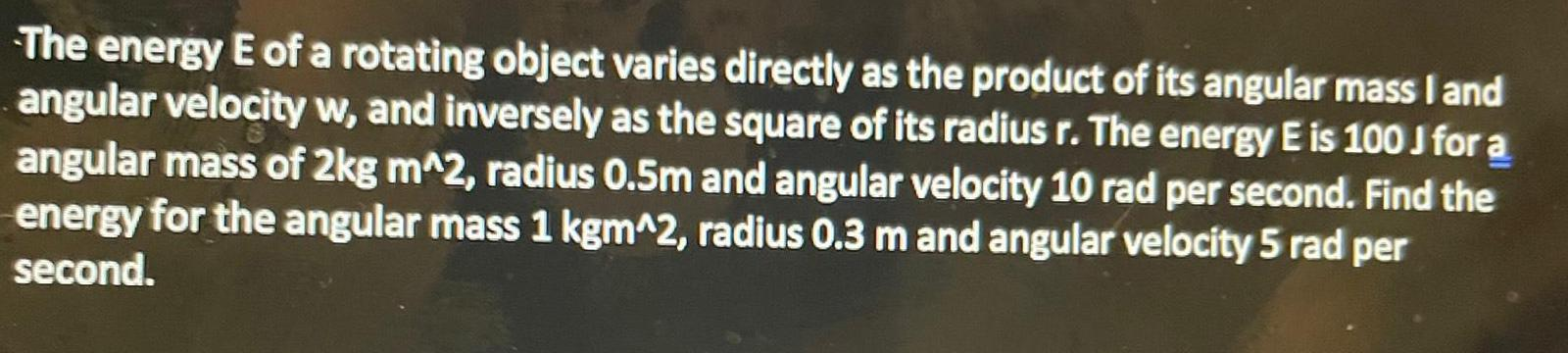Physics
Rotation
The energy E of a rotating object varies directly as the product of its angular mass I and angular velocity w, and inversely as the square of its radius r. The energy E is 100 J for a angular mass of 2kg m^2, radius 0.5m and angular velocity 10 rad per second. Find the energy for the angular mass 1 kgm^2, radius 0.3 m and angular velocity 5 rad per second.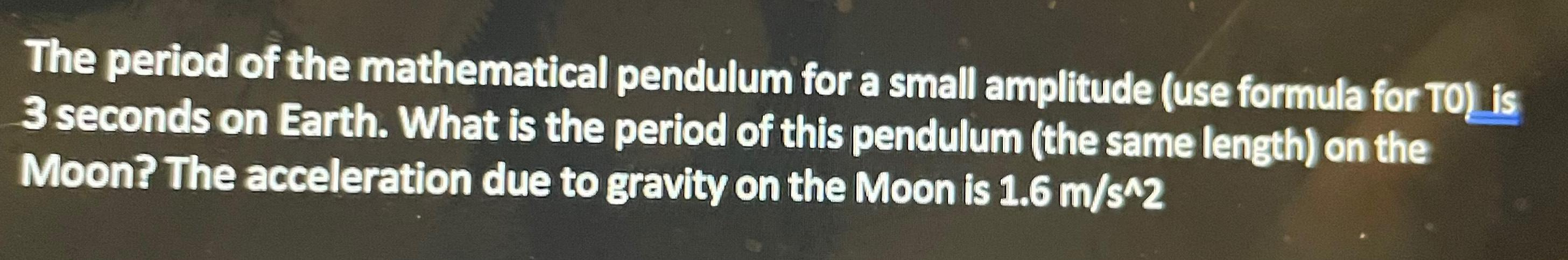Physics
Rotation
The period of the mathematical pendulum for a small amplitude (use formula for T0) is 3 seconds on Earth. What is the period of this pendulum (the same length) on the Moon? The acceleration due to gravity on the Moon is 1.6 m/s^2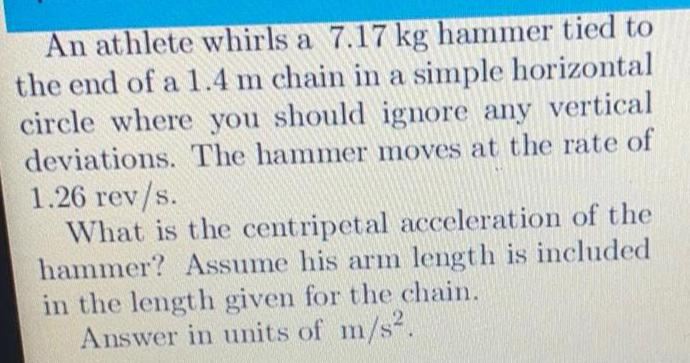Physics
Rotation
An athlete whirls a 7.17 kg hammer tied to the end of a 1.4 m chain in a simple horizontal circle where you should ignore any vertical deviations. The hammer moves at the rate of 1.26 rev/s. What is the centripetal acceleration of the hammer? Assume his arm length is included in the length given for the chain. Answer in units of m/s².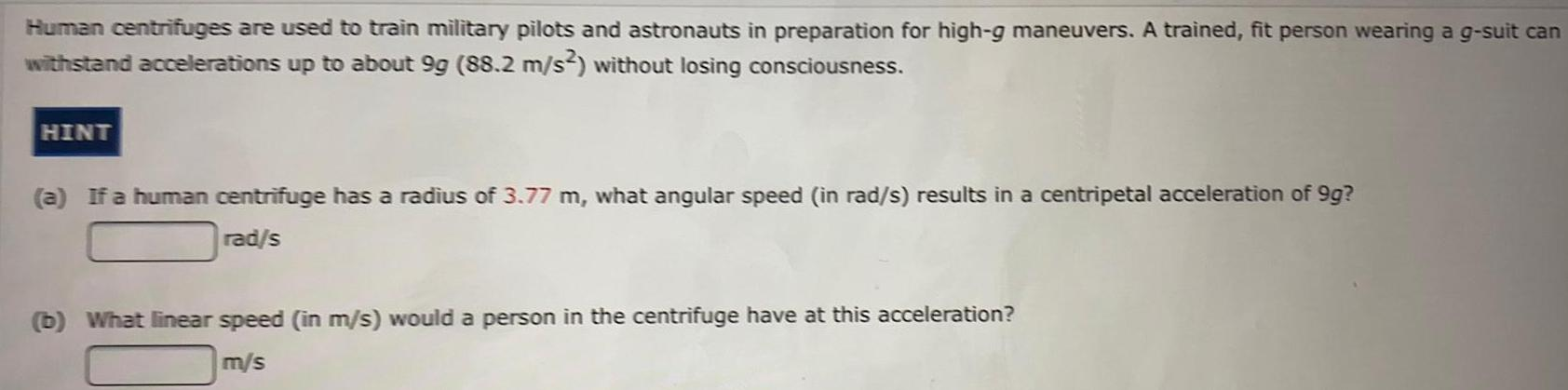Physics
Rotation
Human centrifuges are used to train military pilots and astronauts in preparation for high-g maneuvers. A trained, fit person wearing a g-suit can withstand accelerations up to about 9g (88.2 m/s2) without losing consciousness. (a) If a human centrifuge has a radius of 3.77 m, what angular speed (in rad/s) results in a centripetal acceleration of 9g? rad/s (b) What linear speed (in m/s) would a person in the centrifuge have at this acceleration? m/s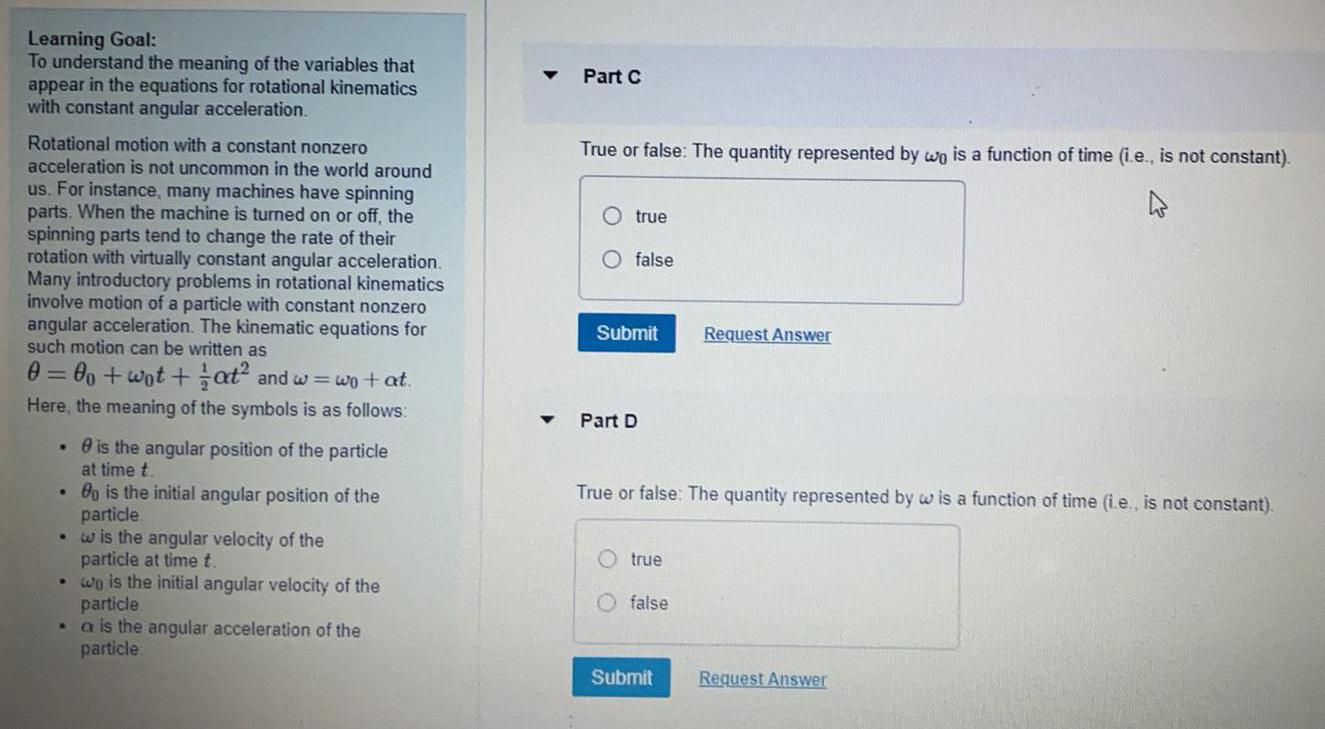Physics
Rotation
Learning Goal: To understand the meaning of the variables that appear in the equations for rotational kinematics with constant angular acceleration. Rotational motion with a constant nonzero acceleration is not uncommon in the world around us. For instance, many machines have spinning parts. When the machine is turned on or off, the spinning parts tend to change the rate of their rotation with virtually constant angular acceleration. Many introductory problems in rotational kinematics involve motion of a particle with constant nonzero angular acceleration. The kinematic equations for such motion can be written as θ = θo+wot+1/2at² and w=wo + at Here, the meaning of the symbols is as follows: θ is the angular position of the particle at time t. . θo is the initial angular position of the particle. w is the angular velocity of the particle at time t. wo is the initial angular velocity of the particle α is the angular acceleration of the particle Part C True or false: The quantity represented by wo is a function of time (i.e., is not constant). true false Part D True or false: The quantity represented by w is a function of time (i.e., is not constant). true false